# 2. [20 pts] Given four-input Boolean functions, F1 (A,B,C,D)-Σ m(4, 5, 10, 11, 12) F 2 (A,B,C,D)m(0, 1, 3, 4, 8, 11...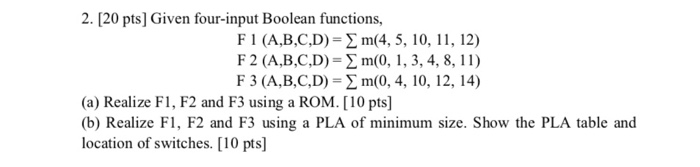2. [20 pts] Given four-input Boolean functions, F1 (A,B,C,D)-Σ m(4, 5, 10, 11, 12) F 2 (A,B,C,D)m(0, 1, 3, 4, 8, 11) F 3 (A,B,C,D) m(0, 4, 10, 12, 14) (a) Realize F1, F2 and F3 using a ROM. [10 pts] (b) Realize F1, F2 and F3 using a PLA of minimum size. Show the PLA table and location of switches. [10 pts]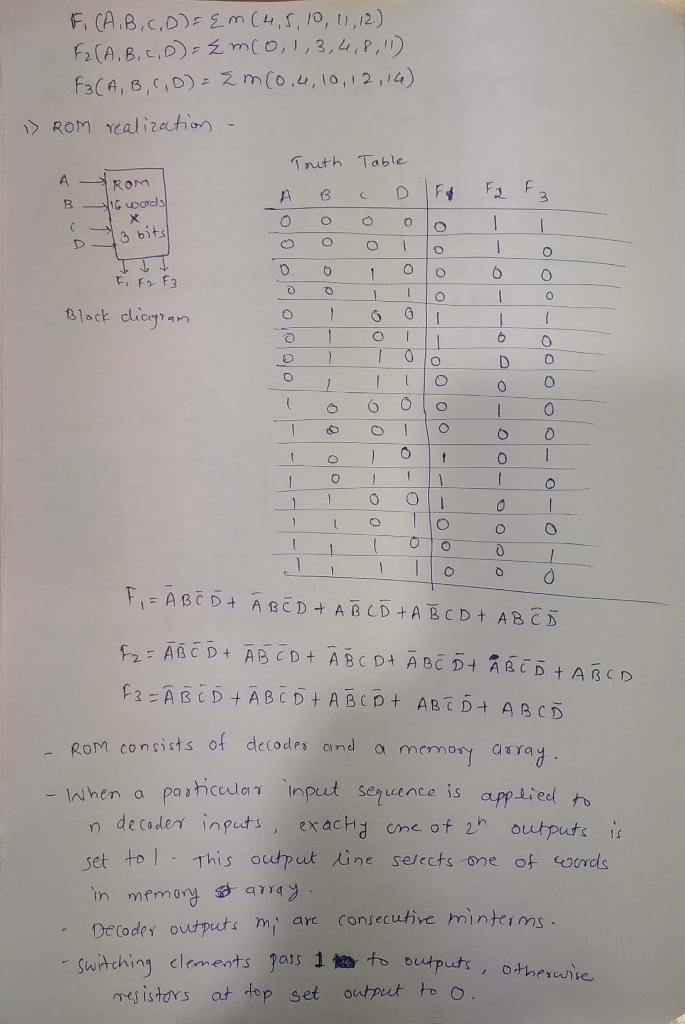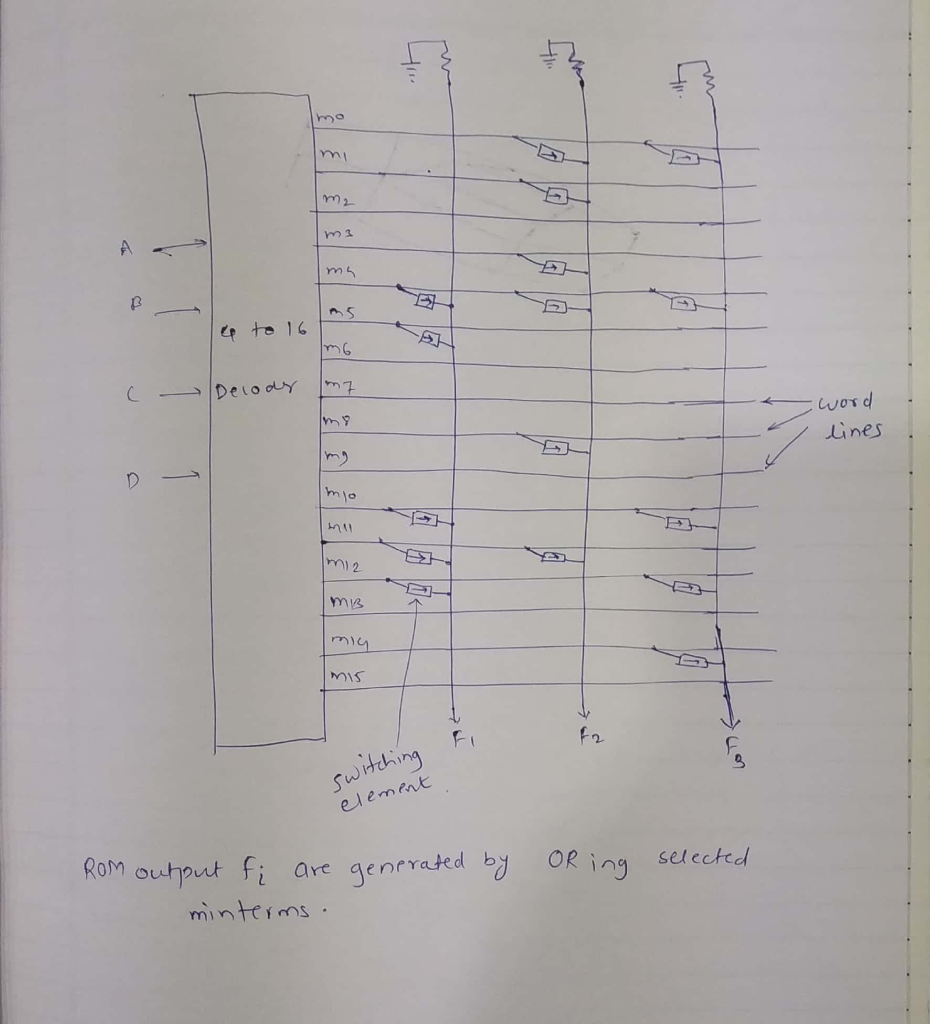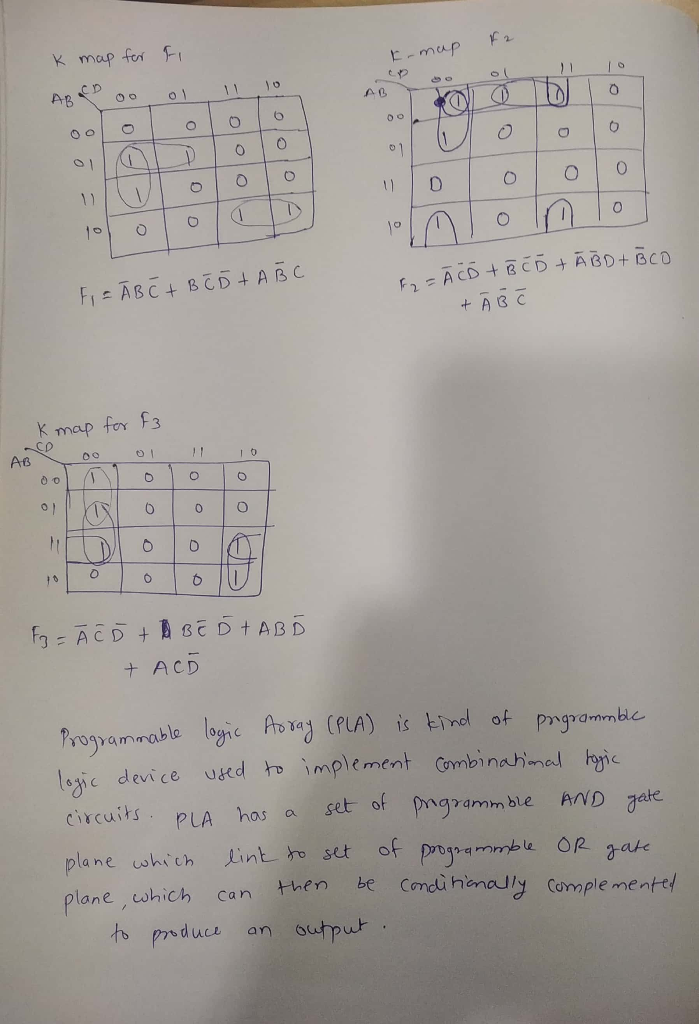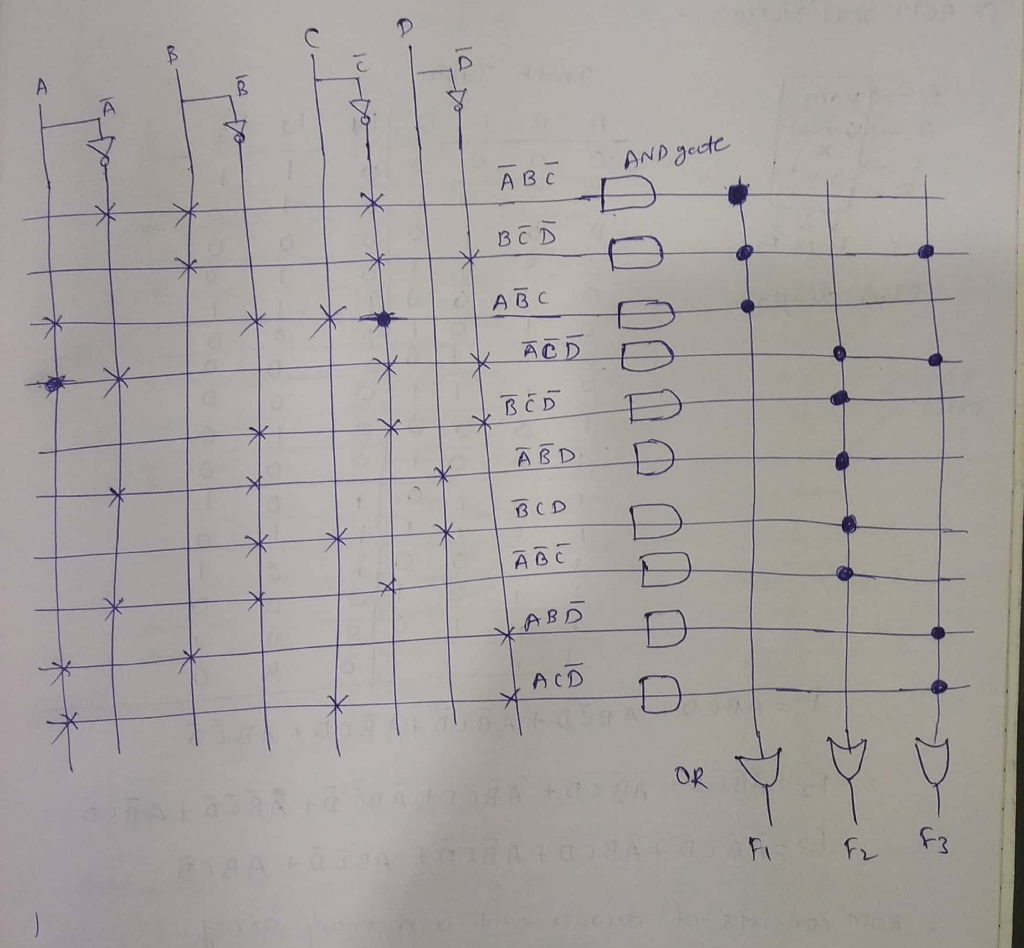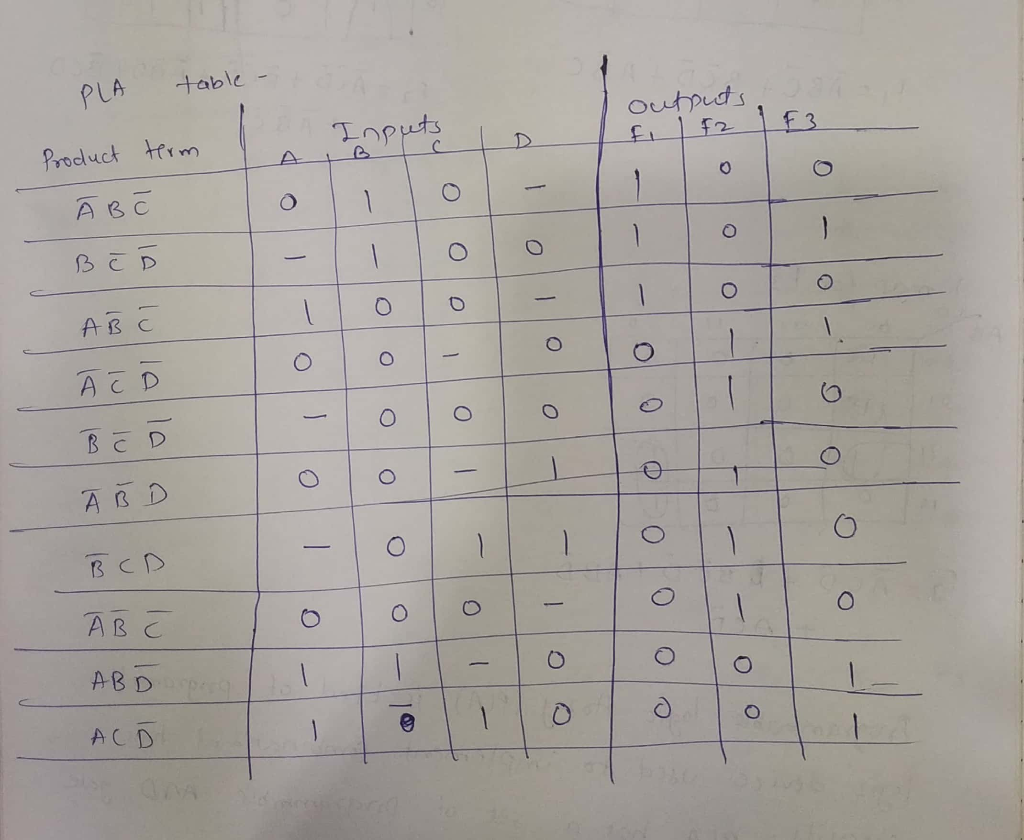##### Add Answer of: 2. [20 pts] Given four-input Boolean functions, F1 (A,B,C,D)-Σ m(4, 5, 10, 11, 12) F 2 (A,B,C,D)m(0, 1, 3, 4, 8, 11...
Similar Homework Help Questions
• ### 2. [20 pts] Given four-input Boolean functions, F1 (A,B,C,D)-Σ m(4, 5, 10, 11, 12) F 2 (A,B,C,D)m(0, 1, 3, 4, 8, 11...2. [20 pts] Given four-input Boolean functions, F1 (A,B,C,D)-Σ m(4, 5, 10, 11, 12) F 2 (A,B,C,D)m(0, 1, 3, 4, 8, 11) F 3 (A,B,C,D) m(0, 4, 10, 12, 14) (a) Realize F1, F2 and F3 using a ROM. [10 pts] (b) Realize F1, F2 and F3 using a PLA of minimum size. Show the PLA table and location of switches. [10 pts] 2. [20 pts] Given four-input Boolean functions, F1 (A,B,C,D)-Σ m(4, 5, 10, 11, 12) F 2 (A,B,C,D)m(0,...

• ### Having the next Boolean functions: F1(x,y,z)-П (1, 3, 5) . F2(x,y,z)-Σ (0, 2, 4, 5) . 1. Make one...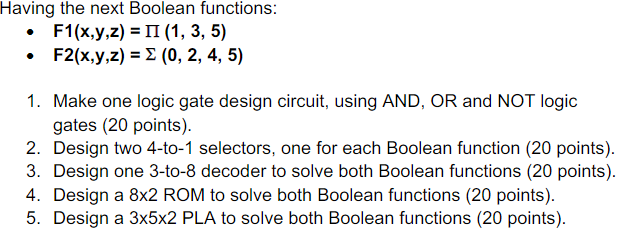Computer architecture Having the next Boolean functions: F1(x,y,z)-П (1, 3, 5) . F2(x,y,z)-Σ (0, 2, 4, 5) . 1. Make one logic gate design circuit, using AND, OR and NOT logic gates (20 points). 2. Design two 4-to-1 selectors, one for each Boolean function (20 points) 3. Design one 3-to-8 decoder to solve both Boolean functions (20 points) 4. Design a 8x2 ROM to solve both Boolean functions (20 points) 5. Design a 3x5x2 PLA to solve both Boolean functions...

• ### The functions are: f1(a,b,c,d) = ∑m(0,1,3,6,9,11) , f2(a,b,c,d) = Πm(2,3,4,5,7,10,11,12,13,14,15) and f3(a,b,c,d) = ab’c’ + a’b’c...

The functions are: f1(a,b,c,d) = ∑m(0,1,3,6,9,11) , f2(a,b,c,d) = Πm(2,3,4,5,7,10,11,12,13,14,15) and f3(a,b,c,d) = ab’c’ + a’b’c +b’cd Complete the following problems for functions f1, f2, and f3 1. Implement the 3 functions on one(1) 4x16 decoder

• ### 4. Express the Boolean functions F as both a sum-of-minterms and a product-of-maxterms 1 0 0...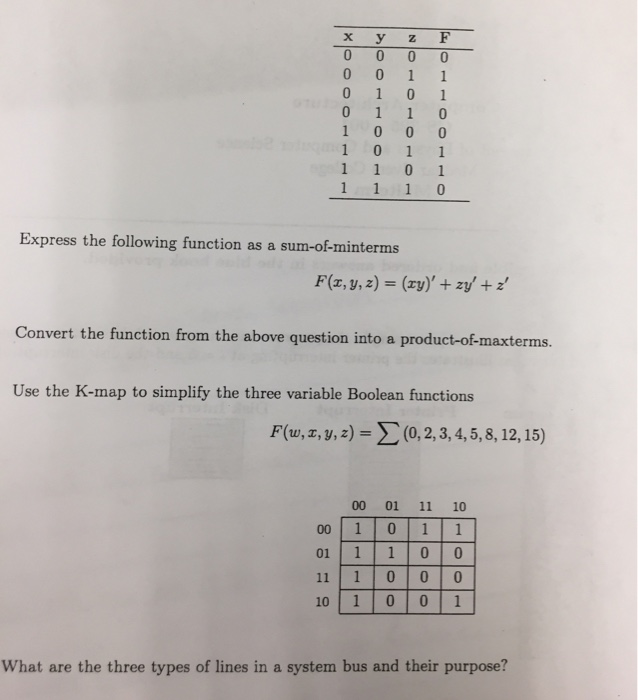4. Express the Boolean functions F as both a sum-of-minterms and a product-of-maxterms 1 0 0 0 Express the following function as a sum-of-minterms F(a, y,z) (zy)' +zy+ Convert the function from the above question into a prodtuct-of macterms Use the K-map to simplify the three variable Boolean functions F(u,x, y, z) = Σ (0, 2, 3, 4, 5, 8, 12, 15) 00 01 11 10 00 10 11 01 1 1 0 0 11 1 0 0 0 10...

• ### (2)A system of three forces and a couple act on a square plate ADEC as shown. 12 kN 20 kNm F 6 kN Evaluate the statical...(2)A system of three forces and a couple act on a square plate ADEC as shown. 12 kN 20 kNm F 6 kN Evaluate the statically equivalent system of forces F, F2 and F3 which act along the sides of the equilateral triangle ABC ( These forces are not proportional to the sides of the triangle.) 650 10 kN reaceses F F. 2 m F2 18.4 kN F1 22.48 k N F2 16.78 kN and F 3 20.7 kN F1...

• ### 1. Read Only Memory Design a ROM that implements the following four Boolean functions: A(x,y,z)-2...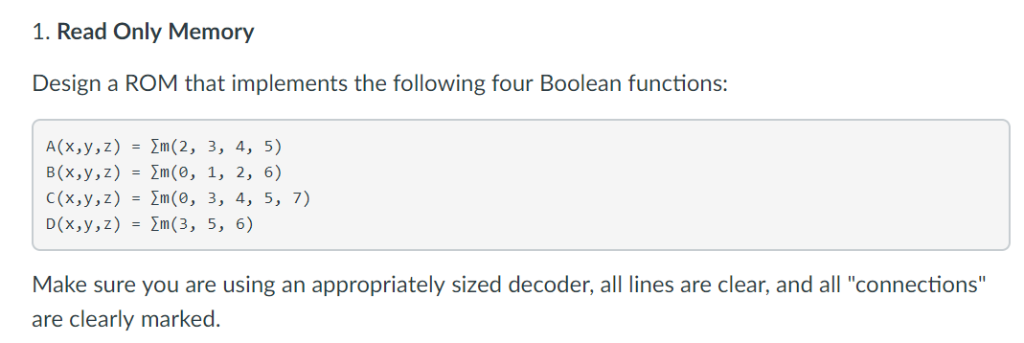1. Read Only Memory Design a ROM that implements the following four Boolean functions: A(x,y,z)-2m(2, 3, 4, 5) B(x,y,z)-2m(0, 1, 2, 6) C(x,y,z) -2m(0, 3, 4, 5, 7) D(x,y,z) -2m(3, 5, 6) Make sure you are using an appropriately sized decoder, all lines are clear, and all "connections" are clearly marked. 1. Read Only Memory Design a ROM that implements the following four Boolean functions: A(x,y,z)-2m(2, 3, 4, 5) B(x,y,z)-2m(0, 1, 2, 6) C(x,y,z) -2m(0, 3, 4, 5, 7) D(x,y,z)...

• ### 7. (24 pts.) Implement the following Boolean function with an 8-to-1 multiplexer, a 2-to-4-line d...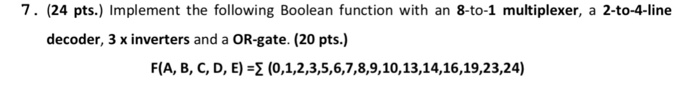7. (24 pts.) Implement the following Boolean function with an 8-to-1 multiplexer, a 2-to-4-line decoder, 3 x inverters and a OR-gate. (20 pts.) F(A, B, C, D, E) -2 (0,1,2,3,5,6,7,8,9,10,13,14,16,19,23,24) 7. (24 pts.) Implement the following Boolean function with an 8-to-1 multiplexer, a 2-to-4-line decoder, 3 x inverters and a OR-gate. (20 pts.) F(A, B, C, D, E) -2 (0,1,2,3,5,6,7,8,9,10,13,14,16,19,23,24)

• ### C++ CODE /* This is program project 2 on page 695. * Before you begin the...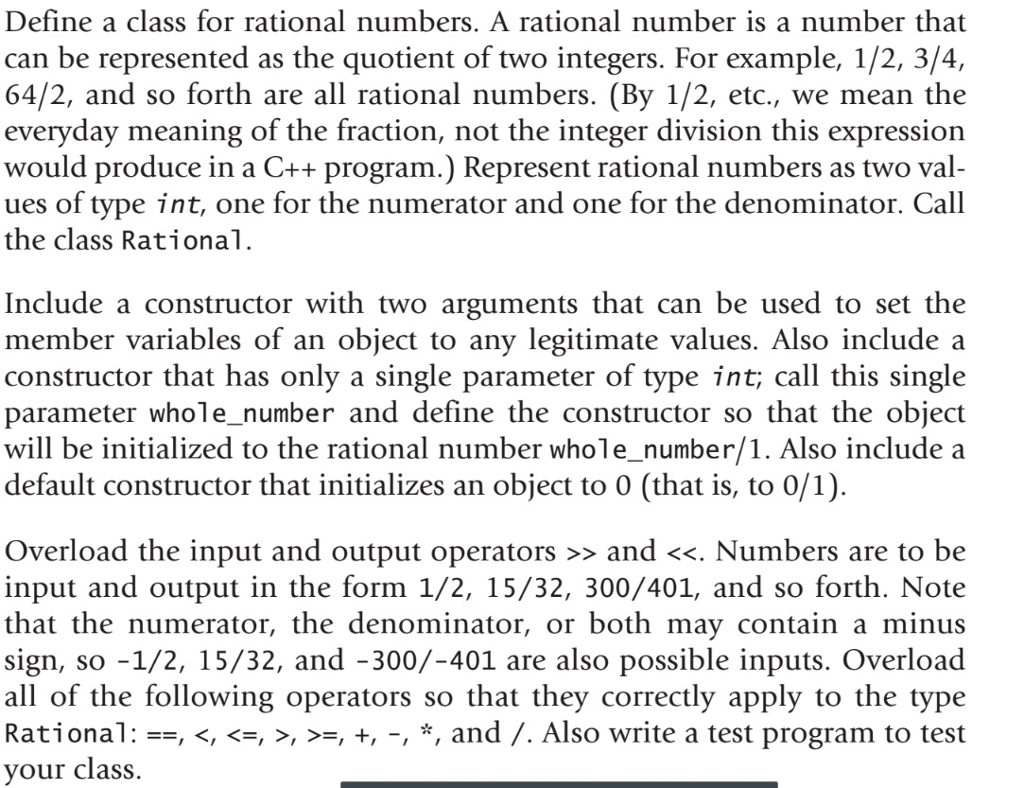C++ CODE /* This is program project 2 on page 695. * Before you begin the project, please read the project description * on page 695 first. * * Author: Your Name * Version: Dates */ #include <iostream> #include <cmath> #include <cassert> using namespace std; class Fraction { public: // constructor Fraction(int a, int b); // generate a fraction which is a/b Fraction(int a); // generate a fraction which is a/1 Fraction(); // generate a fraction which is 0/1. i.e...

• ### using these alphabets a,b,c,d e,f spell these letters a=1,b=2,c=3,d=4,e=5,f=e 2+5+4=11 bed 2+5+1+4=12 bead 6+5+5+4=20 feed what numbers and alphabet = 30

using these alphabets a,b,c,d e,f spell these letters a=1,b=2,c=3,d=4,e=5,f=e 2+5+4=11 bed 2+5+1+4=12 bead 6+5+5+4=20 feed what numbers and alphabet = 30

• ### using these alphabets a,b,c,d e,f spell these letters a=1,b=2,c=3,d=4,e=5,f=6 2+5+4=11 bed 2+5+1+4=12 bead 6+5+5+4=20 feed what numbers and alphabet = 30

using these alphabets a,b,c,d e,f spell these letters a=1,b=2,c=3,d=4,e=5,f=6 2+5+4=11 bed 2+5+1+4=12 bead 6+5+5+4=20 feed what numbers and alphabet = 30

Need Online Homework Help?